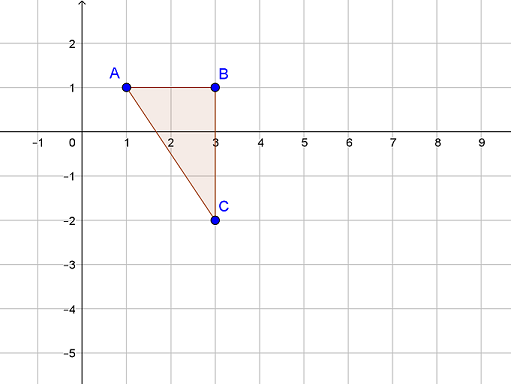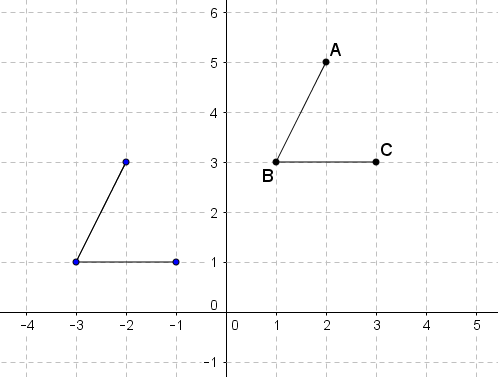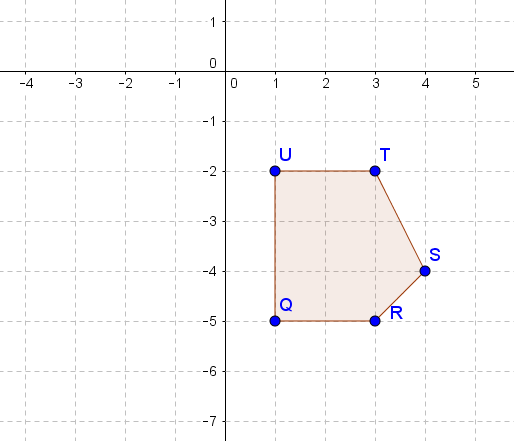Match Fishtank is now Fishtank Learning!

# Transformations and Angle Relationships

## Objective

Describe and apply properties of translations. Use coordinate points to represent relationships between translated figures.

## Common Core Standards

### Core Standards

?

• 8.G.A.1.A — Lines are taken to lines, and line segments to line segments of the same length.

• 8.G.A.1.B — Angles are taken to angles of the same measure.

• 8.G.A.1.C — Parallel lines are taken to parallel lines.

• 8.G.A.2 — Understand that a two-dimensional figure is congruent to another if the second can be obtained from the first by a sequence of rotations, reflections, and translations; given two congruent figures, describe a sequence that exhibits the congruence between them.

• 8.G.A.3 — Describe the effect of dilations, translations, rotations, and reflections on two-dimensional figures using coordinates.

## Criteria for Success

?

1. Apply properties of translations to reason about features of translated figures; for example, knowing that translations preserve side length, reason that areas and perimeters of figures are also preserved.
2. Determine coordinate points of translated vertices.
3. Given coordinate points, determine the translation that took place between two congruent figures.

## Tips for Teachers

?

• This is the second lesson on translations. In the first lesson students understand some of the features that are preserved in translations; in this lesson, students extend on that understanding and reason about other features such as perimeter, angle measure, area, etc. (MP.2).
• The following materials are useful for this lesson: patty paper (or transparency paper), graph paper, protractors. Students may use any of these tools to verify congruency between two figures or equivalence between two measures (MP.5).

#### Remote Learning Guidance

If you need to adapt or shorten this lesson for remote learning, we suggest prioritizing Anchor Problems 1 and 2 (benefit from worked examples). Find more guidance on adapting our math curriculum for remote learning here.

#### Fishtank Plus

• Problem Set
• Student Handout Editor
• Vocabulary Package

## Anchor Problems

?

### Problem 1

Triangle ${ABC}$ is translated 3 units down and 5 units to the right to create triangle ${A’B’C’}$.1. What is the length of ${\overline{B'C'}}$?
2. Which statement is true?
1. The perimeter of${ABC}$ is equal to the perimeter of ${{{{A'B'C'}}}}$, but the areas of the two triangles are different.
2. The perimeter of ${ABC}$ is equal to the perimeter of ${{{{A'B'C'}}}}$, and the areas of the two triangles are the same.
3. The area of ${ABC}$ is equal to the area of ${{{{A'B'C'}}}}$, but the perimeters of the two triangles are different.
4. Both the areas and perimeters of ${ABC}$ and ${{{{A'B'C'}}}}$ are different.

### Problem 2

Angle ${ABC}$ is translated as shown below. Use the translation to answer the questions that follow.1. Describe the translation and name the new angle.
2. Will ${\overleftrightarrow{AB}}$ and ${\overleftrightarrow{A'B'}}$ ever intersect? How do you know?
3. If $\angle {ABC}$ is ${70^{\circ}}$, what is the measure of ${\angle A'B'C'}$? How do you know?

### Problem 3

Below are the coordinate points from an original figure and a translated figure.

$A(1,2) \rightarrow A' (-1, -1)$

$B(-1,4) \rightarrow B'(-3,1)$

$C(5,4) \rightarrow C' (3,1)$

Describe the translation that occurred.

## Problem Set

?The following resources include problems and activities aligned to the objective of the lesson that can be used to create your own problem set.

• Examples where students are given a figure and description of translation, name information about coordinate points of translated figure, and identify features that are the same and/or different between two figures (similar to target task)
• Examples where students are given coordinate points and name the translation that is described (similar to Anchor Problem #3)
• Examples where students perform translations
• Examples where students are given two figures and determine if one figure can be mapped onto the other using a translation (i.e., are the two figures congruent?)

?

Figure ${QRSTU}$ undergoes a translation that takes it 4 units left and 2 units down. Use the diagram to answer the questions that follow.1. What are the coordinates of point ${T'}$?
2. What are the coordinates of point ${Q'}$?
3.  Name two line segments in the translated figure that will be parallel to ${\overline{UT}}$.
4. Name three features that will be the same in the original figure and the translated figure.
5. Name one feature that will be different in the original figure and the translated figure.

?